# Lecture 5

## Welcome!

• All the prior weeks have presented you with the fundamental building blocks of programming.
• All you have learned in C will enable you to implement these building blocks in higher-level programming languages such as Python.
• Today, we are going to talk about organizing data in memory.

## Data Structures

• Data structures essentially are forms of organization in memory.
• There are many ways to organize data in memory.
• Abstract data structures are those that we can conceptually imagine. When learning about computer science, it’s often useful to begin with these conceptual data structures. Learning these will make it easier later to understand how to implement more concrete data structures.

## Stacks and Queues

• Queues are one form of abstract data structure.
• Queues have specific properties. Namely, they are FIFO or “first in first out.” You can imagine yourself in a line for a ride at an amusement park. The first person in the line gets to go on the ride first. The last person gets to go on the ride last.
• Queues have specific actions associated with them. For example, an item can be enqueued; that is, the item can join the line or queue. Further, an item can be dequeued or leave the queue once it reaches the front of the line.
• Queues contrast a stack. Fundamentally, the properties of a stack are different than a queue. Specifically, it is LIFO or “last in first out.” Just like stacking trays in a cafeteria, a tray that is placed in a stack last is the first that may be picked up.
• Stacks have specific actions associated with them. For example, push places something on top of a stack. Pop is removing something from the top of the stack.
• In code, you might imagine a stack as follows:

const int CAPACITY = 50;

typedef struct
{
person people[CAPACITY];
int size;
}
stack;


Notice that an array called people is of type person. The CAPACITY is how high the stack could be. The integer size is how full the stack actually is, regardless of how much it could hold.

• You might imagine that the above code has a limitation. Since the capacity of the array is always predetermined in this code. Therefore, the stack may always be oversized. You might imagine only using one place in the stack out of 5000.
• It would be nice for our stack to be dynamic – able to grow as items are added to it.

## Resizing Arrays

• Rewinding to Week 2, we introduced you to your first data structure.
• An array is a block of contiguous memory.
• You might imagine an array as follows: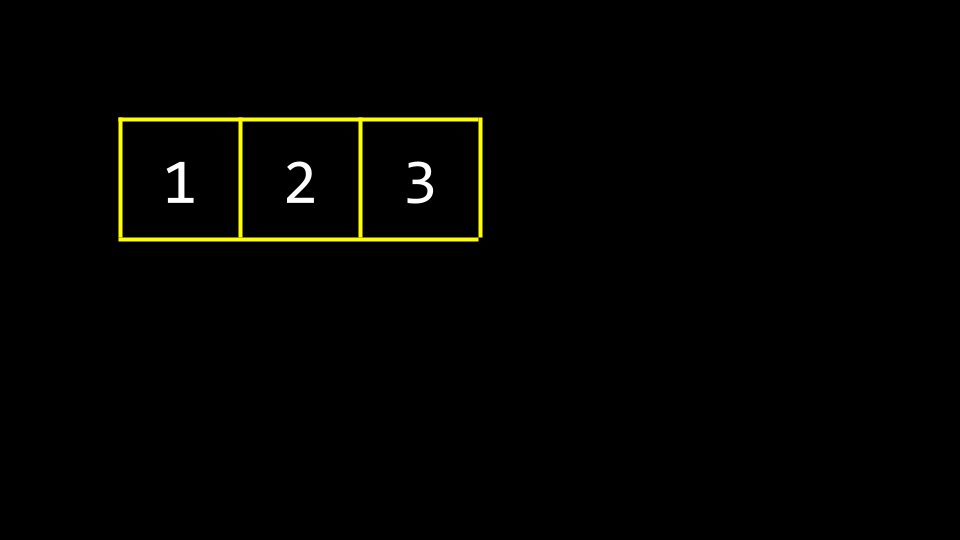• In memory, there are other values being stored by other programs, functions, and variables. Many of these may be unused garbage values that were utilized at one point but are available now for use.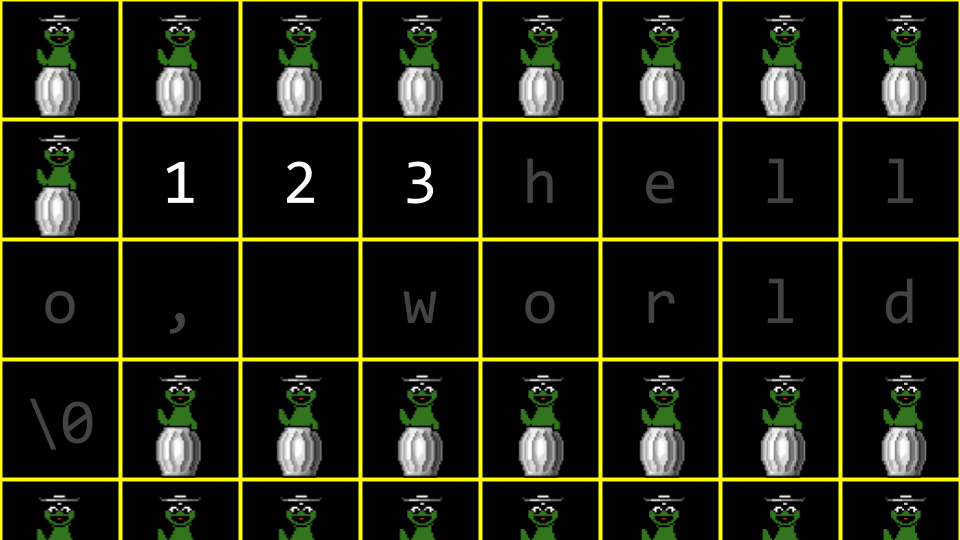• Imagine you wanted to store a fourth value 4 in our array? What would be needed is to allocate a new area of memory and move the old array to a new one.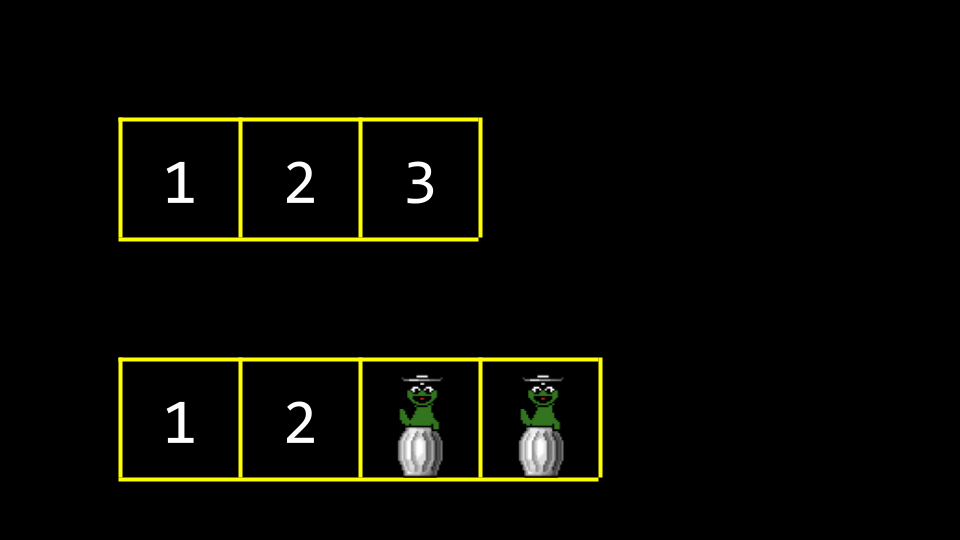• Old garbage values would be overwritten with our new data.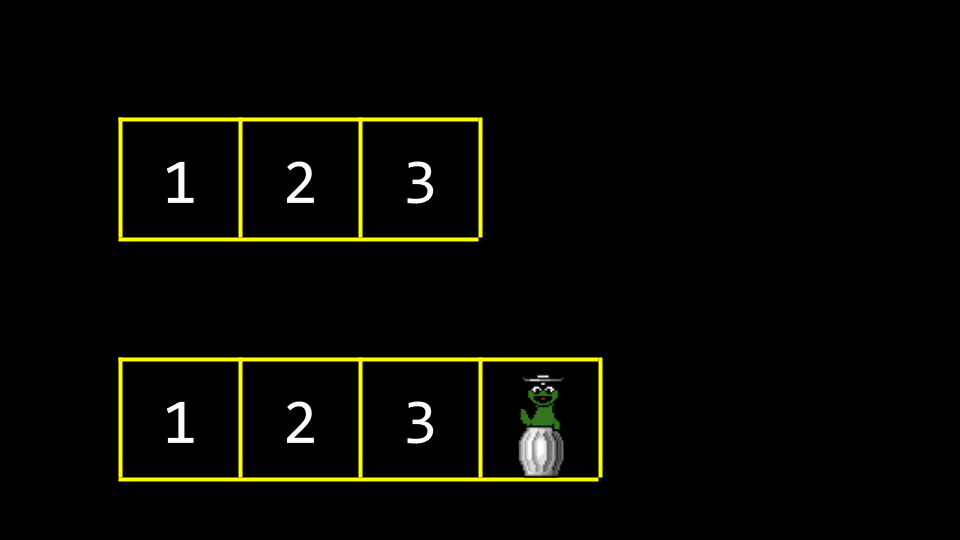• One of the drawbacks of this approach is that it’s bad design: Every time we add a number, we have to copy the array item by item.

• Wouldn’t it be nice if we were able to put the 4 somewhere else in memory? By definition, this would no longer be an array because 4 would no longer be in contiguous memory.

• In your terminal, type code list.c and write code as follows:

// Implements a list of numbers with an array of fixed size

#include <stdio.h>

int main(void)
{
// List of size 3
int list;

// Initialize list with numbers
list = 1;
list = 2;
list = 3;

// Print list
for (int i = 0; i < 3; i++)
{
printf("%i\n", list[i]);
}
}


Notice that the above is very much like what we learned earlier in this course. We have memory being preallocated for three items.

• Building upon our knowledge obtained more recently, we can leverage our understanding of pointers to create a better design in this code. Modify your code as follows:

// Implements a list of numbers with an array of dynamic size

#include <stdio.h>
#include <stdlib.h>

int main(void)
{
// List of size 3
int *list = malloc(3 * sizeof(int));
if (list == NULL)
{
return 1;
}

// Initialize list of size 3 with numbers
list = 1;
list = 2;
list = 3;

// List of size 4
int *tmp = malloc(4 * sizeof(int));
if (tmp == NULL)
{
free(list);
return 1;
}

// Copy list of size 3 into list of size 4
for (int i = 0; i < 3; i++)
{
tmp[i] = list[i];
}

// Add number to list of size 4
tmp = 4;

// Free list of size 3
free(list);

// Remember list of size 4
list = tmp;

// Print list
for (int i = 0; i < 4; i++)
{
printf("%i\n", list[i]);
}

// Free list
free(list);
return 0;


Notice that a list of size three integers is created. Then, three memory addresses can be assigned the values 1, 2, and 3. Then, a list of size four is created. Next, the list is copied from the first to the second. The value for the 4 is added to the tmp list. Since the block of memory that list points to is no longer used, it is freed using the command free(list). Finally, the compiler is told to point list pointer now to the block of memory that tmp points to. The contents of list are printed and then freed.

• It’s useful to think about list and tmp as both signs that point at a chunk of memory. As in the example above, list at one point pointed to an array of size 3. By the end, list was told to point to a chunk of memory of size 4. Technically, by the end of the above code, tmp and list both pointed to the same block of memory.

• C comes with a very useful function called realloc that will reallocate the memory for you. realloc takes two arguments. First, it asks you to specify the array you are attempting to copy. Second, it asks you to specify the size to which you would like the final array to be. Modify your code as follows:

// Implements a list of numbers with an array of dynamic size using realloc

#include <stdio.h>
#include <stdlib.h>

int main(void)
{
// List of size 3
int *list = malloc(3 * sizeof(int));
if (list == NULL)
{
return 1;
}

// Initialize list of size 3 with numbers
list = 1;
list = 2;
list = 3;

// Resize list to be of size 4
int *tmp = realloc(list, 4 * sizeof(int));
if (tmp == NULL)
{
free(list);
return 1;
}
list = tmp;

list = 4;

// Print list
for (int i = 0; i < 4; i++)
{
printf("%i\n", list[i]);
}

// Free list
free(list);
return 0;
}


Notice that int *tmp = realloc(list, 4 * sizeof(int)) creates a list of size four integers. Then, it copies the values of list to this new array. Finally, a pointer called tmp points to the location of memory of this new array. The copying is handled by realloc. Once that copy is made, the memory at the location of list is freed. Then, the pointer called list is pointed at the location of tmp, where the new array is located.

• You can imagine how using realloc for a queue or stack could be useful. As the amount of data grows, realloc could be used to grow or shrink the stack or queue.

• In recent weeks, you have learned about three useful primitives. A struct is a data type that you can define yourself. A . in dot notation allows you to access variables inside that structure. The * operator is used to declare a pointer or dereference a variable.
• Today, you are introduced to the -> operator. It is an arrow. This operator goes to an address and looks inside of a structure.
• A Linked list is one of the most powerful data structures within C. A linked list allows you to include values that are located at varying areas of memory. Further, they allow you to dynamically grow and shrink the list as you desire.
• You might imagine three values stored at three different areas of memory as follows: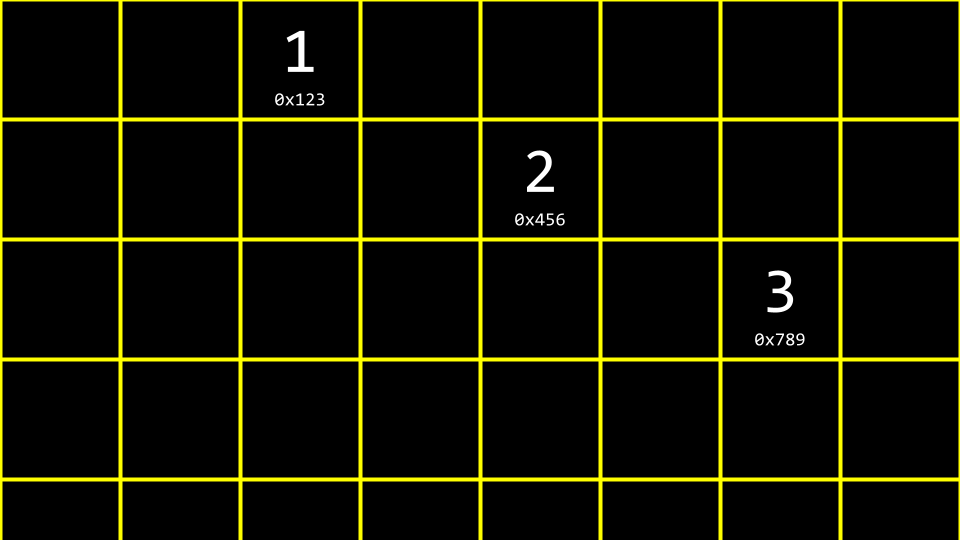• How could one stitch together these values in a list?
• We could imagine this data pictured above as follows: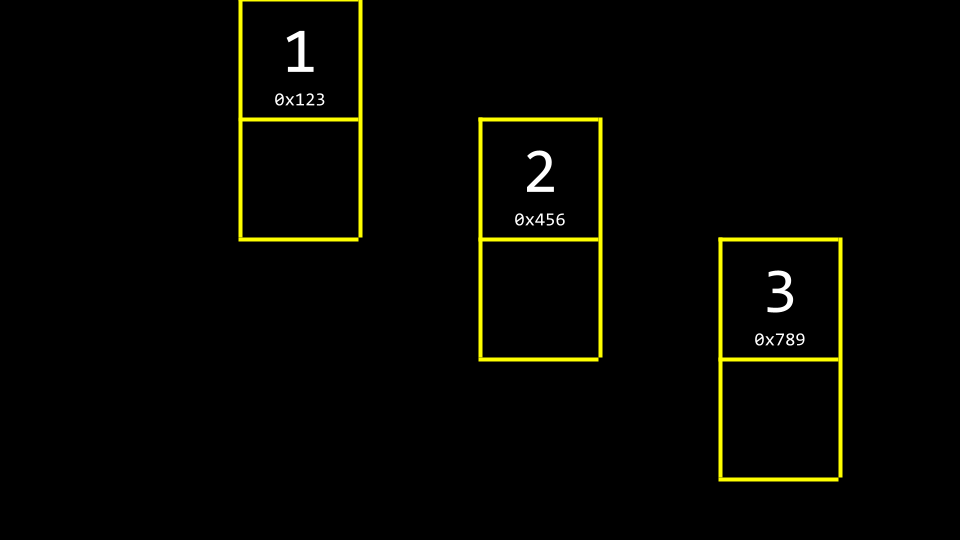• We could utilize more memory to keep track of where the next item is.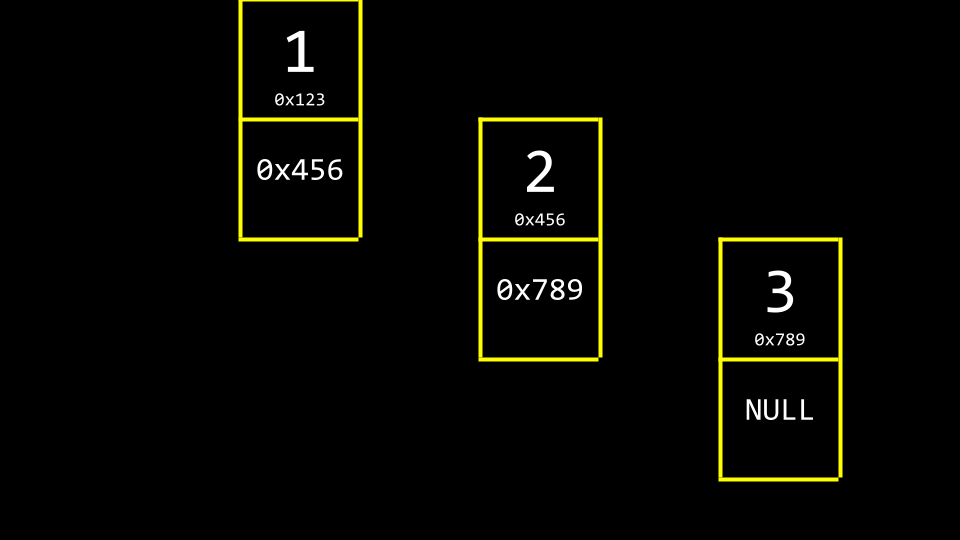Notice that NULL is utilized to indicate that nothing else is next in the list.

• By convention, we would keep one more element in memory, a pointer, that keeps track of the first item in the list.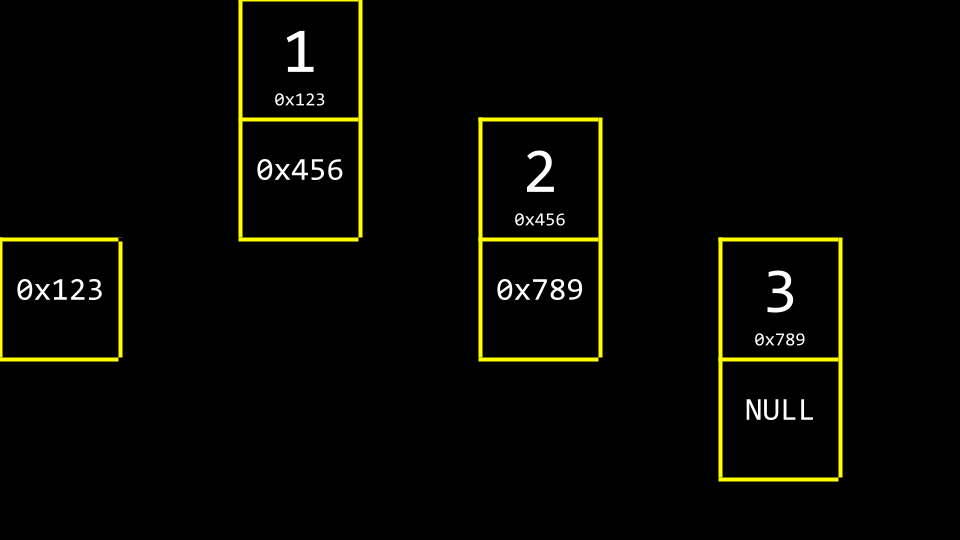• Abstracting away the memory addresses, the list would appear as follows: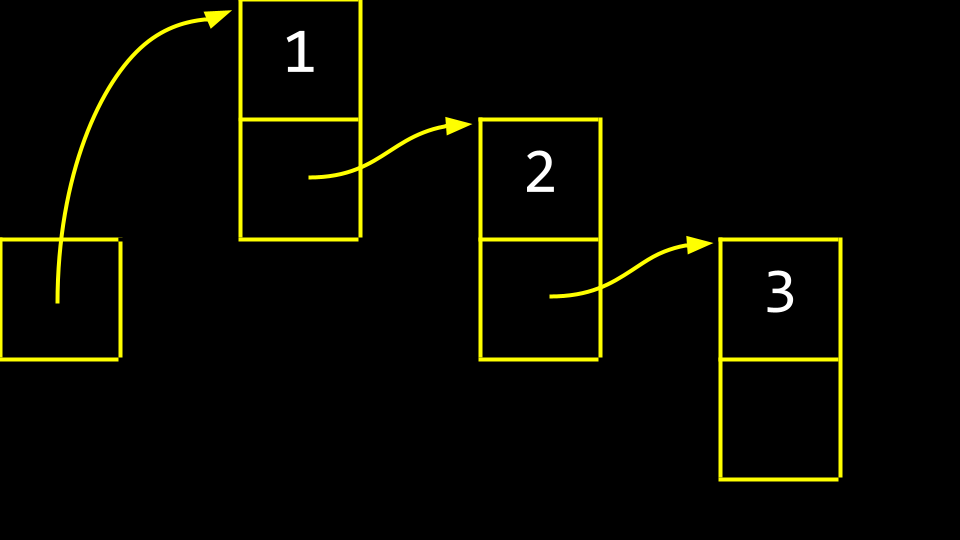• These boxes are called nodes. A node contains both an item and a pointer called next. In code, you can imagine a node as follows:

typedef struct node
{
int number;
struct node *next;
}
node;


Notice that the item contained within this node is an integer called number. Second, a pointer to a node called next is included, which will point to another node somewhere in memory.

• Linked lists are not stored in a contiguous block of memory. They can grow as large as you wish, provided that enough system resources exist. The downside, however, is that more memory is required to keep track of the list instead of an array. This is because for each element, you must store not just the value of the element, but also a pointer to the next node. Further, linked lists cannot be indexed into like is possible in an array because we need to pass through the first $$n - 1$$ elements to find the location of the $$n$$th element. Because of this, the list pictured above must be linearly searched. Binary search, therefore, is not possible in a list constructed as above.

• Conceptually, we can imagine the process of creating a linked list. First, node *list is declared, but it is of a garbage value.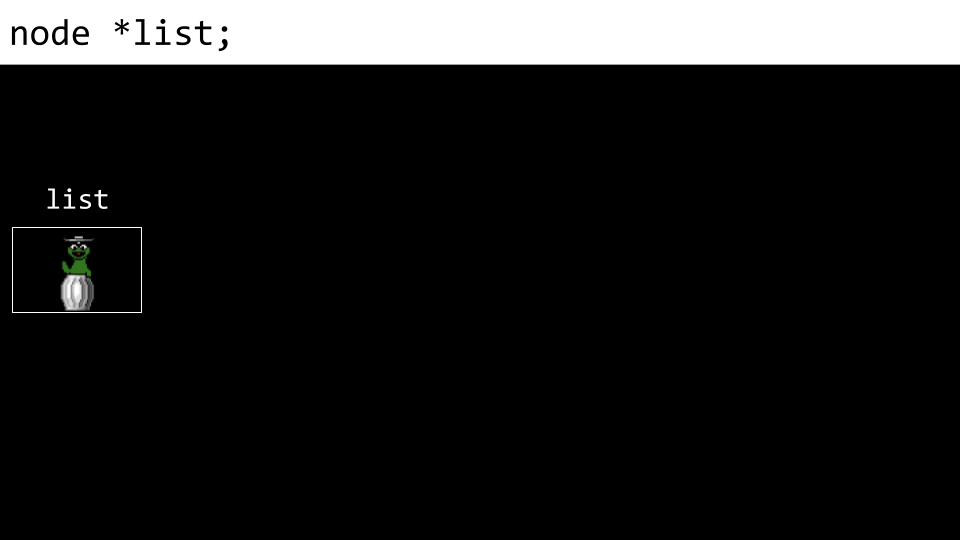• Next, a node called n is allocated in memory.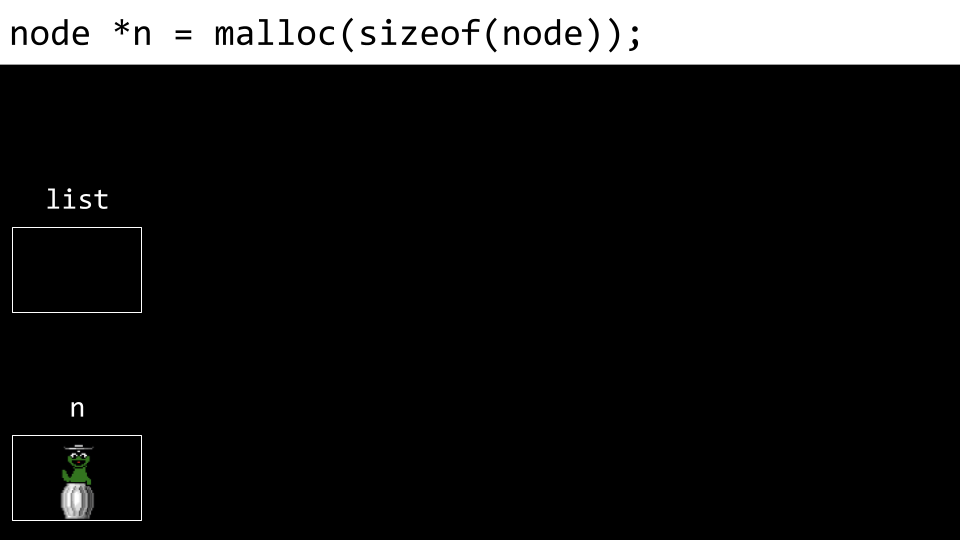• Next, the number of node is assigned the value 1.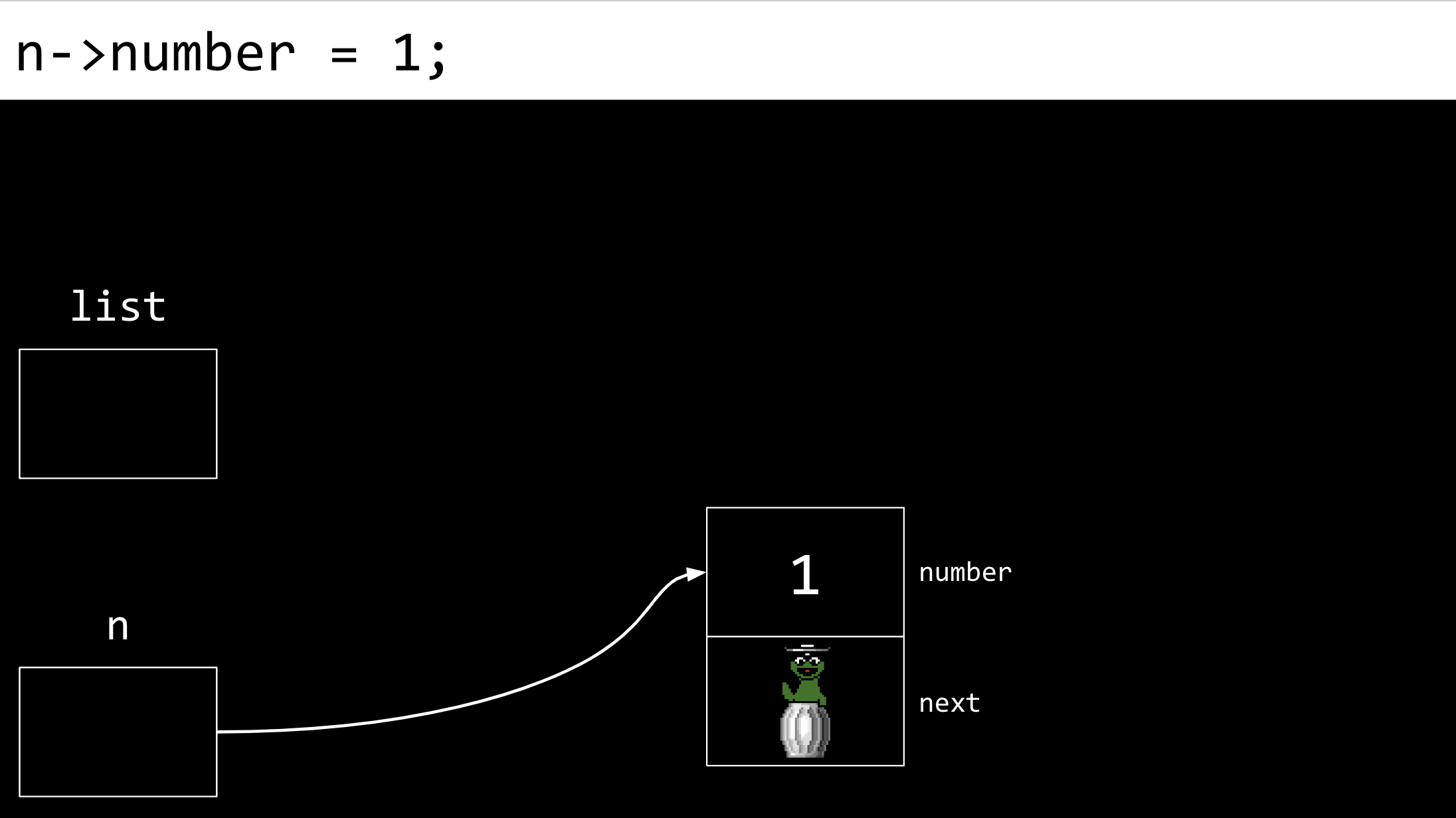• Next, the node’s next field is assigned NULL.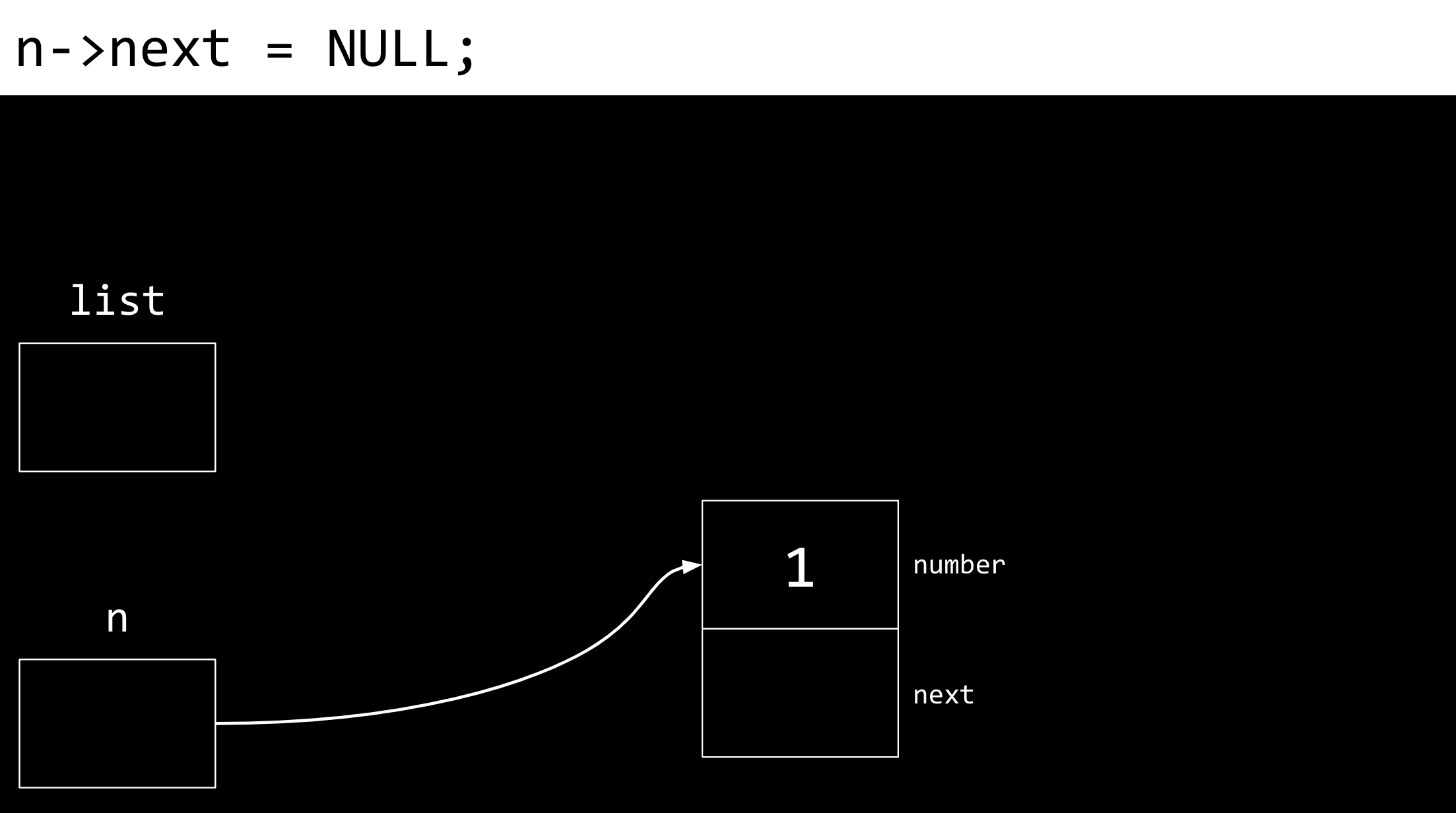• Next, list is pointed at the memory location to where n points. n and list now point to the same place.• A new node is then created. Both the number and next field are both filled with garbage values.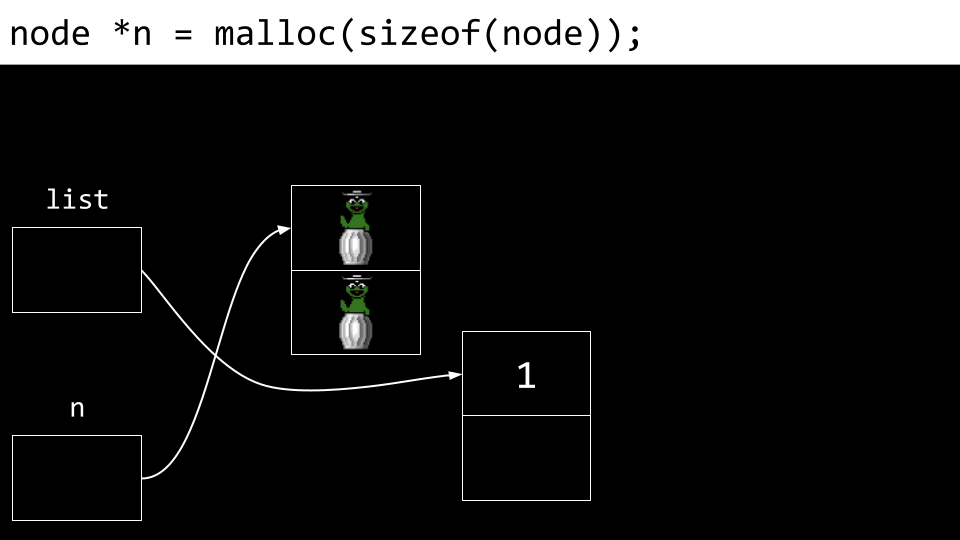• The number value of n’s node (the new node) is updated to 2.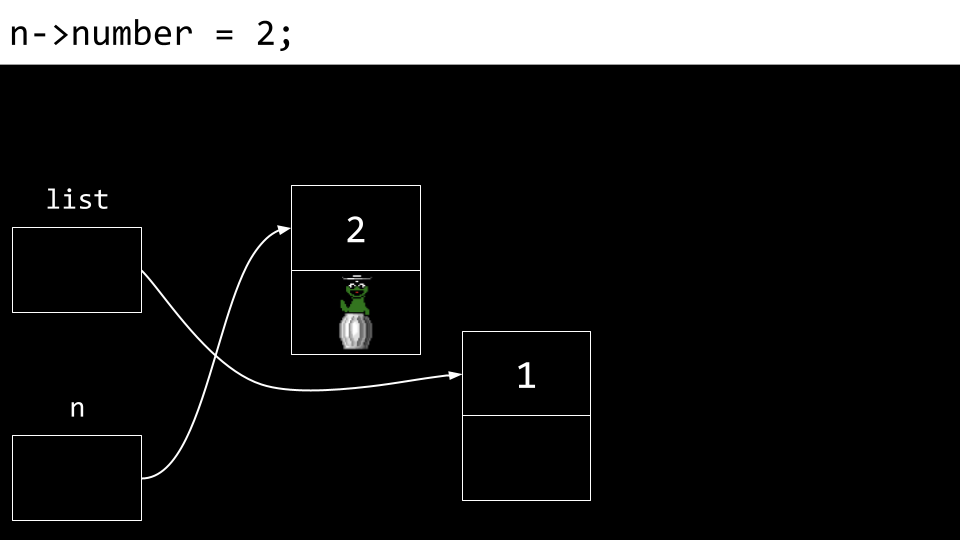• Also, the next field is updated as well.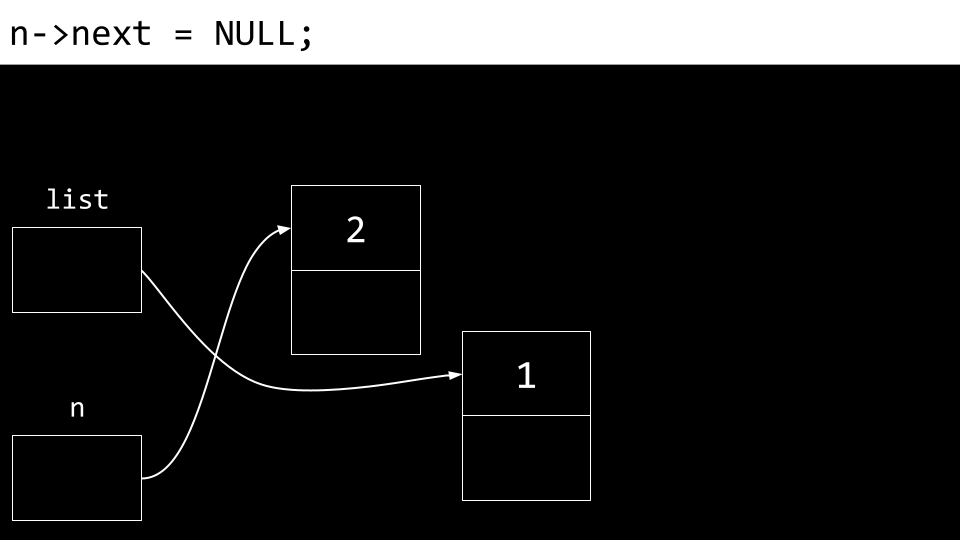• Most important, we do not want to lose our connection to any of these nodes lest they be lost forever. Accordingly, n’s next field is pointed to the same memory location as list.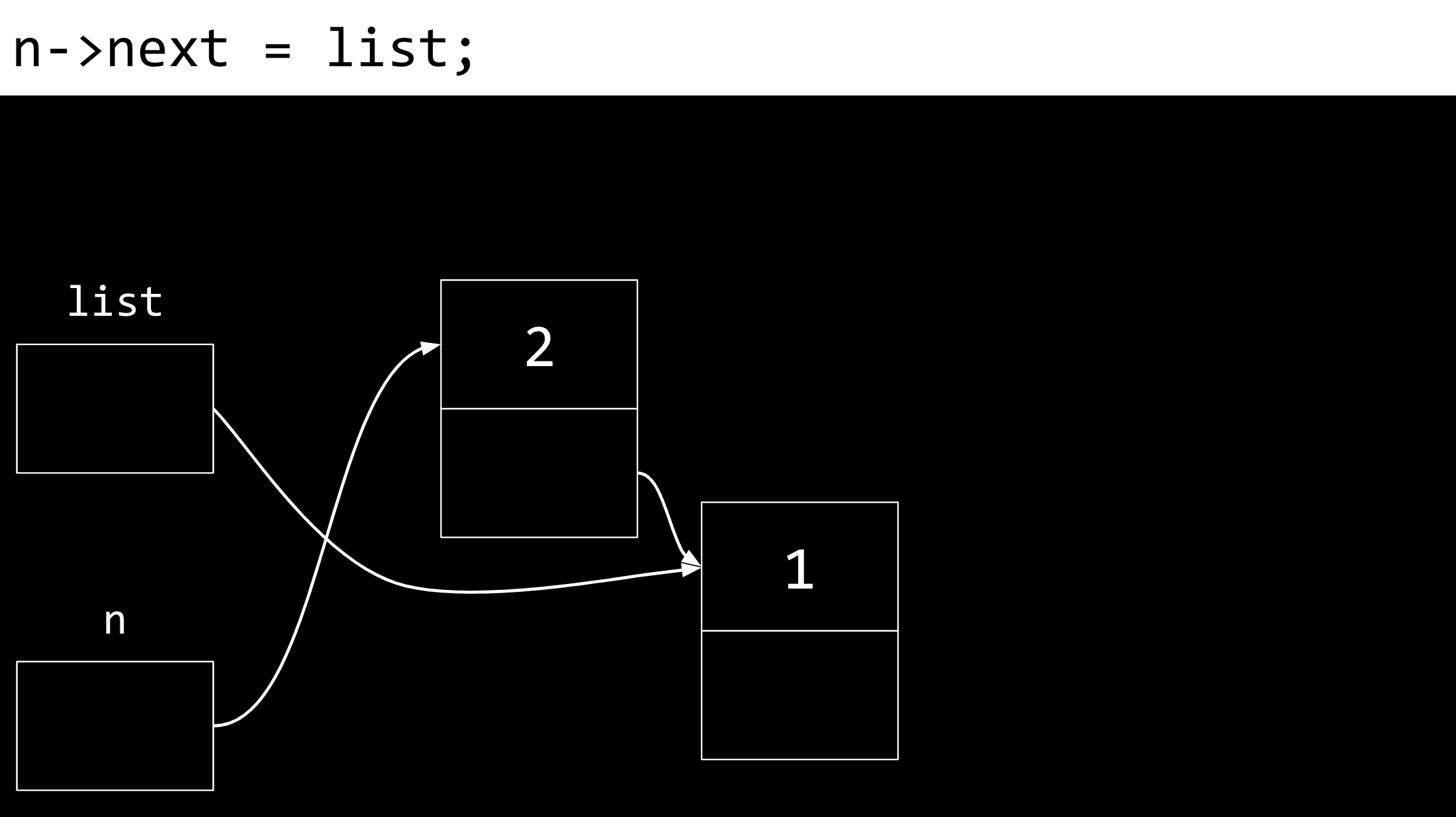• Finally, list is updated to point at n. We now have a linked list of two items.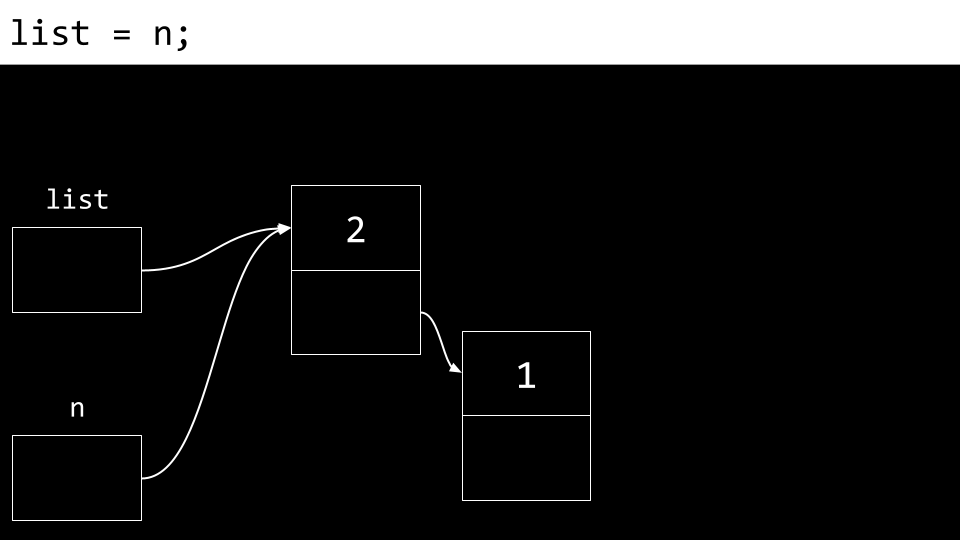• To implement this in code, modify your code as follows:

// Prepends numbers to a linked list, using while loop to print

#include <cs50.h>
#include <stdio.h>
#include <stdlib.h>

typedef struct node
{
int number;
struct node *next;
}
node;

int main(int argc, char *argv[])
{
// Memory for numbers
node *list = NULL;

// For each command-line argument
for (int i = 1; i < argc; i++)
{
// Convert argument to int
int number = atoi(argv[i]);

// Allocate node for number
node *n = malloc(sizeof(node));
if (n == NULL)
{
return 1;
}
n->number = number;
n->next = NULL;

// Prepend node to list
n->next = list;
list = n;
}

// Print numbers
node *ptr = list;
while (ptr != NULL)
{
printf("%i\n", ptr->number);
ptr = ptr->next;
}

// Free memory
ptr = list;
while (ptr != NULL)
{
node *next = ptr->next;
free(ptr);
ptr = next;
}
}


Notice that what the user inputs at the command line is put into the number field of a node called n, and then that node is added to the list. For example, ./list 1 2 will put the number 1 into the number field of a node called n, then put a pointer to list into the next field of the node, and then update list to point to n. That same process is repeated for 2. Next, node *ptr = list creates a temporary variable that points at the same spot that list points to. The while prints what at the node ptr points to, and then updates ptr to point to the next node in the list. Finally, all the memory is freed.

• Considering the amount of time required to search this list, it is in the order of $$O(n)$$, as in the worst case the entire list must always be searched to find an item. The time complexity for adding a new element to the list will depend on where that element is added. This is illustrated in the examples below.
• You, as the programmer, have the choice of how to implement your list. The following code, for example, implements a linked list that prepends numbers to the front of the list:

// Prepends numbers to a linked list, using for loop to print

#include <cs50.h>
#include <stdio.h>
#include <stdlib.h>

typedef struct node
{
int number;
struct node *next;
}
node;

int main(int argc, char *argv[])
{
// Memory for numbers
node *list = NULL;

// For each command-line argument
for (int i = 1; i < argc; i++)
{
// Convert argument to int
int number = atoi(argv[i]);

// Allocate node for number
node *n = malloc(sizeof(node));
if (n == NULL)
{
return 1;
}
n->number = number;
n->next = NULL;

// Prepend node to list
n->next = list;
list = n;
}

// Print numbers
for (node *ptr = list; ptr != NULL; ptr = ptr->next)
{
printf("%i\n", ptr->number);
}

// Free memory
node *ptr = list;
while (ptr != NULL)
{
node *next = ptr->next;
free(ptr);
ptr = next;
}
}


Notice how numbers are placed at the start of the list. This insertion will run in the order of $$O(1)$$, as the number of steps it takes to do this does not depend on the size of the list.

• Further, you could place numbers at the end of the list as illustrated in this code:

// Implements a list of numbers using a linked list

#include <cs50.h>
#include <stdio.h>
#include <stdlib.h>

typedef struct node
{
int number;
struct node *next;
}
node;

int main(int argc, char *argv[])
{
// Memory for numbers
node *list = NULL;

// For each command-line argument
for (int i = 1; i < argc; i++)
{
// Convert argument to int
int number = atoi(argv[i]);

// Allocate node for number
node *n = malloc(sizeof(node));
if (n == NULL)
{
return 1;
}
n->number = number;
n->next = NULL;

// If list is empty
if (list == NULL)
{
// This node is the whole list
list = n;
}

// If list has numbers already
else
{
// Iterate over nodes in list
for (node *ptr = list; ptr != NULL; ptr = ptr->next)
{
// If at end of list
if (ptr->next == NULL)
{
// Append node
ptr->next = n;
break;
}
}
}
}

// Print numbers
for (node *ptr = list; ptr != NULL; ptr = ptr->next)
{
printf("%i\n", ptr->number);
}

// Free memory
node *ptr = list;
while (ptr != NULL)
{
node *next = ptr->next;
free(ptr);
ptr = next;
}
}


Notice how this code walks down this list to find the end. When appending an element, (adding to the end of the list) our code will run in $$O(n)$$, as we have to go through our entire list before we can add the final element.

// Implements a sorted list of numbers using a linked list

#include <cs50.h>
#include <stdio.h>
#include <stdlib.h>

typedef struct node
{
int number;
struct node *next;
}
node;

int main(int argc, char *argv[])
{
// Memory for numbers
node *list = NULL;

// For each command-line argument
for (int i = 1; i < argc; i++)
{
// Convert argument to int
int number = atoi(argv[i]);

// Allocate node for number
node *n = malloc(sizeof(node));
if (n == NULL)
{
return 1;
}
n->number = number;
n->next = NULL;

// If list is empty
if (list == NULL)
{
list = n;
}

// If number belongs at beginning of list
else if (n->number < list->number)
{
n->next = list;
list = n;
}

// If number belongs later in list
else
{
// Iterate over nodes in list
for (node *ptr = list; ptr != NULL; ptr = ptr->next)
{
// If at end of list
if (ptr->next == NULL)
{
// Append node
ptr->next = n;
break;
}

// If in middle of list
if (n->number < ptr->next->number)
{
n->next = ptr->next;
ptr->next = n;
break;
}
}
}
}

// Print numbers
for (node *ptr = list; ptr != NULL; ptr = ptr->next)
{
printf("%i\n", ptr->number);
}

// Free memory
node *ptr = list;
while (ptr != NULL)
{
node *next = ptr->next;
free(ptr);
ptr = next;
}
}


Notice how this list is sorted as it is built. To insert an element in this specific order, our code will still run in $$O(n)$$ for each insertion, as in the worst case we will have to look through all current elements.

## Trees

• Binary search trees are another data structure that can be used to store data more efficiently such that it can be searched and retrieved.
• You can imagine a sorted sequence of numbers.• Imagine then that the center value becomes the top of a tree. Those that are less than this value are placed to the left. Those values that are more than this value are to the right.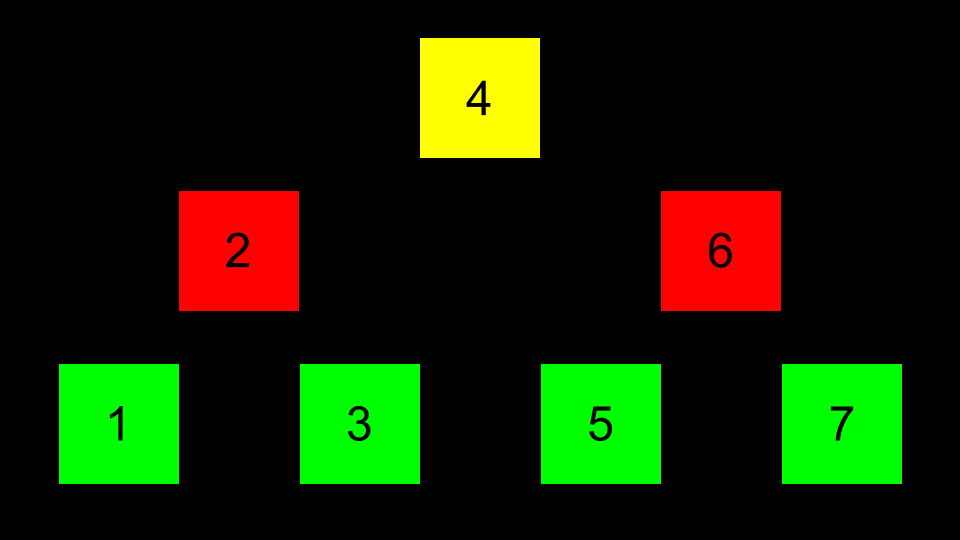• Pointers can then be used to point to the correct location of each area of memory such that each of these nodes can be connected.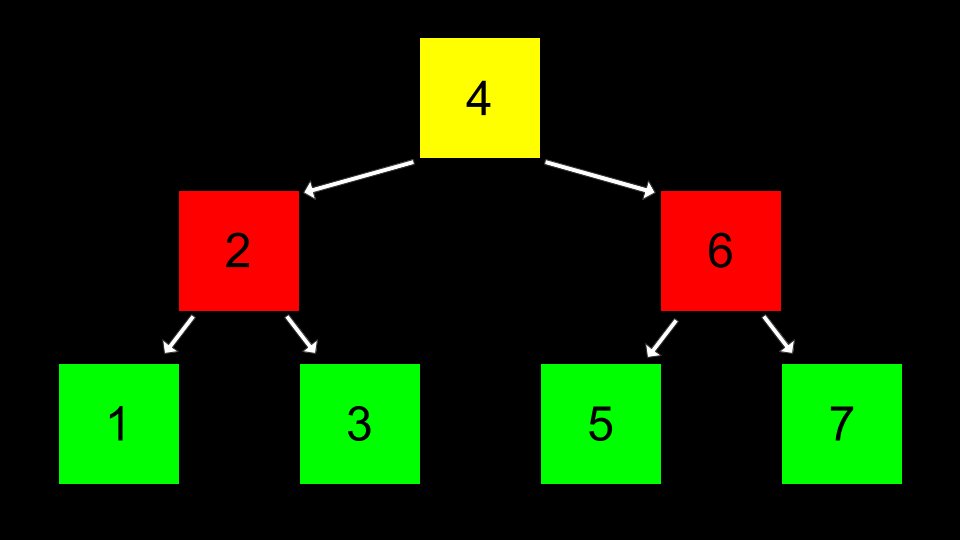• In code, this can be implemented as follows.

// Implements a list of numbers as a binary search tree

#include <stdio.h>
#include <stdlib.h>

// Represents a node
typedef struct node
{
int number;
struct node *left;
struct node *right;
}
node;

void free_tree(node *root);
void print_tree(node *root);

int main(void)
{
// Tree of size 0
node *tree = NULL;

node *n = malloc(sizeof(node));
if (n == NULL)
{
return 1;
}
n->number = 2;
n->left = NULL;
n->right = NULL;
tree = n;

n = malloc(sizeof(node));
if (n == NULL)
{
free_tree(tree);
return 1;
}
n->number = 1;
n->left = NULL;
n->right = NULL;
tree->left = n;

n = malloc(sizeof(node));
if (n == NULL)
{
free_tree(tree);
return 1;
}
n->number = 3;
n->left = NULL;
n->right = NULL;
tree->right = n;

// Print tree
print_tree(tree);

// Free tree
free_tree(tree);
return 0;
}

void free_tree(node *root)
{
if (root == NULL)
{
return;
}
free_tree(root->left);
free_tree(root->right);
free(root);
}

void print_tree(node *root)
{
if (root == NULL)
{
return;
}
print_tree(root->left);
printf("%i\n", root->number);
print_tree(root->right);
}

• Searching this tree could be implemented as follows:

bool search(node *tree, int number)
{
if (tree == NULL)
{
return false;
}
else if (number < tree->number)
{
return search(tree->left, number);
}
else if (number > tree->number)
{
return search(tree->right, number);
}
else if (number == tree->number)
{
return true;
}
}


Notice this search function begins by going to the location of tree. Then, it uses recursion to search for number.

• A tree like the above offers dynamism that an array does not offer. It can grow and shrink as we wish.

## Dictionaries

• Dictionaries are another data structure.
• Dictionaries, like actual book-form dictionaries that have a word and a definition, have a key and a value.
• The holy grail of time complexity is $$O(1)$$ or constant time. That is, the ultimate is for access to be instantaneous.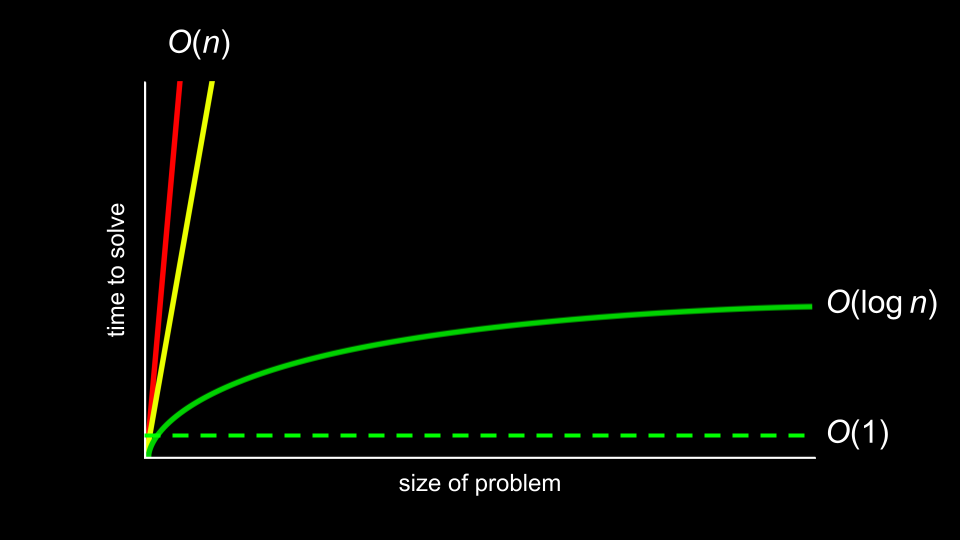• Dictionaries can offer this speed of access.

## Hashing and Hash Tables

• Hashing is the idea of taking a value and being able to output a value that becomes a shortcut to it later.
• For example, hashing apple may hash as a value of 1, and berry may be hashed as 2. Therefore, finding apple is as easy as asking the hash algorithm where apple is stored. While not ideal in terms of design, ultimately, putting all a’s in one bucket and b’s in another, this concept of bucketizing hashed values illustrates how you can use this concept: a hashed value can be used to shortcut finding such a value.
• A hash function is an algorithm that reduces a larger value to something small and predictable. Generally, this function takes in an item you wish to add to your hash table, and returns an integer representing the array index in which the item should be placed.
• A hash table is a fantastic combination of both arrays and linked lists. When implemented in code, a hash table is an array of pointers to nodes.
• A hash table could be imagined as follows:Notice that this is an array that is assigned each value of the alphabet.

• Then, at each location of the array, a linked list is used to track each value being stored there: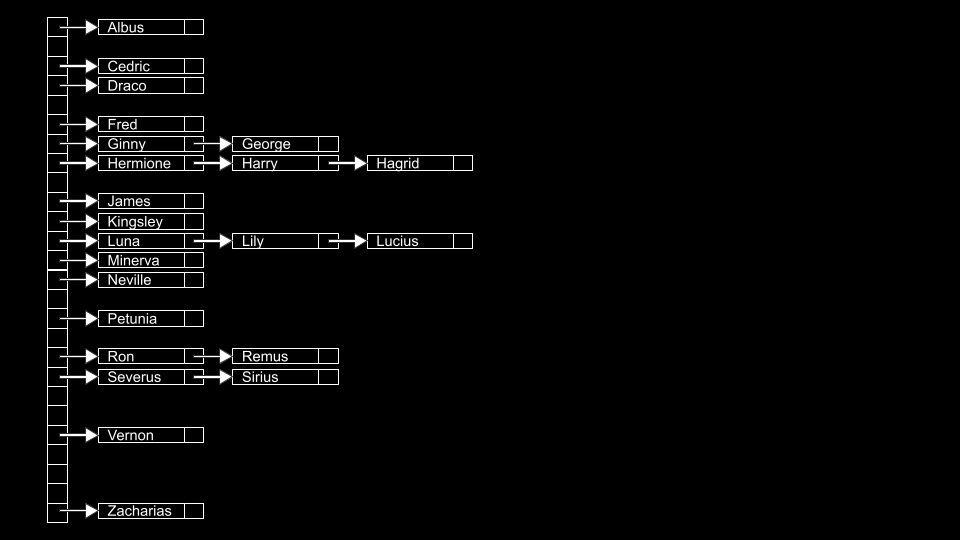• Collisions are when you add values to the hash table, and something already exists at the hashed location. In the above, collisions are simply appended to the end of the list.
• Collisions can be reduced by better programming your hash table and hash algorithm. You can imagine an improvement upon the above as follows: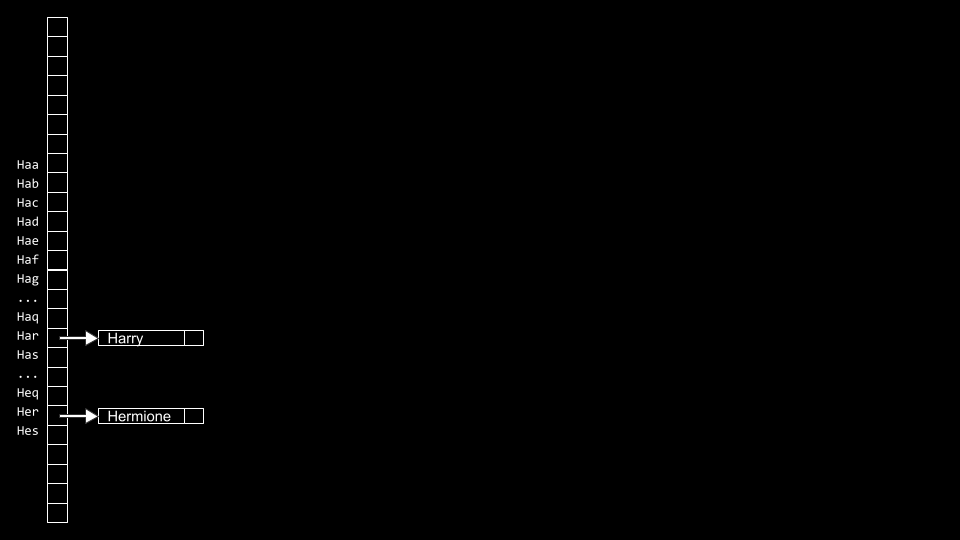• You, as the programmer, have to make a decision about the advantages of using more memory to have a large hash table and potentially reducing search time or using less memory and potentially increasing search time.

## Tries

• Tries are another form of data structure.
• Tries are always searchable in constant time.
• One downside to Tries is that they tend to take up a large amount of memory. Notice that we need $$26 \times 5 = 130$$ nodes just to store Hagrid!
• Hagrid would be stored as follows: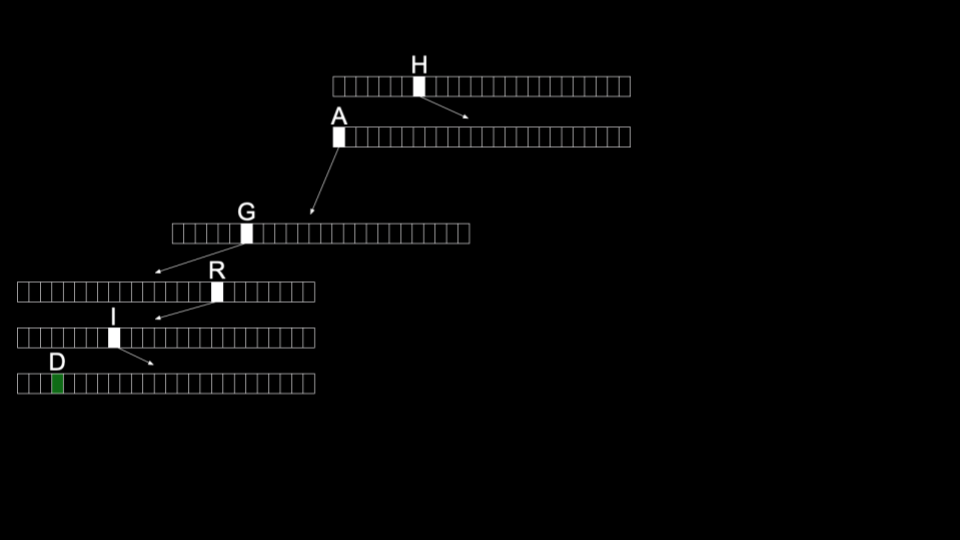• Harry would then be stored as follows: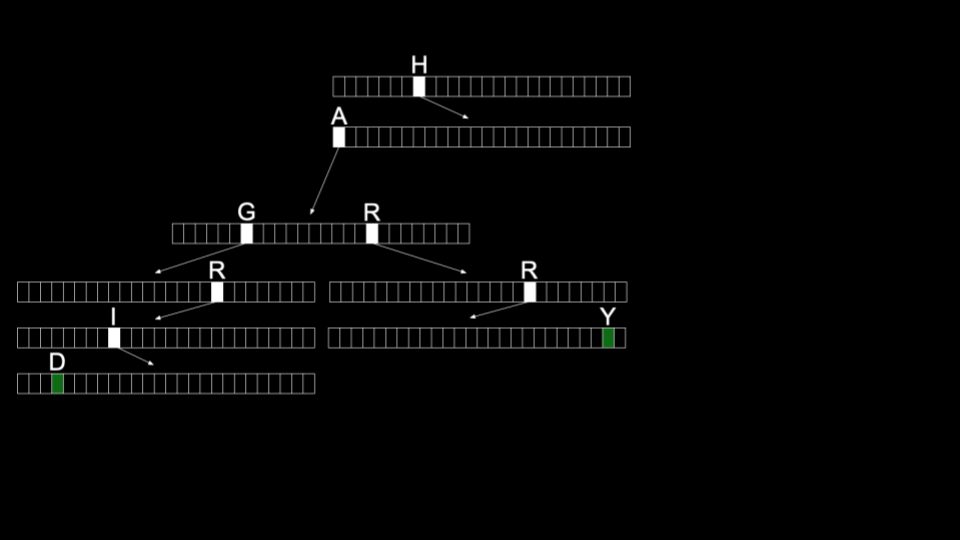## Summing Up

In this lesson, you learned about using pointers to build new data structures. Specifically, we delved into…

• Data structures
• Stacks and queues
• Resizing arrays